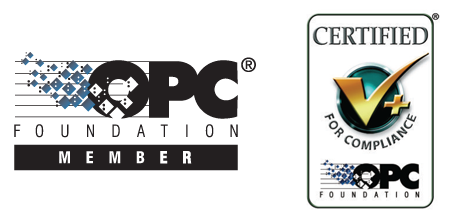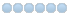## Professional OPC Development Tools# Online Forums

Technical support is provided through Support Forums below. Anybody can view them; you need to Register/Login to our site (see links in upper right corner) in order to Post questions. You do not have to be a licensed user of our product.

Please read Rules for forum posts before reporting your issue or asking a question. OPC Labs team is actively monitoring the forums, and replies as soon as possible. Various technical information can also be found in our Knowledge Base. For your convenience, we have also assembled a Frequently Asked Questions page.

Do not use the Contact page for technical issues.

# PHP - receiving NULLs for all data

24 Jul 2018 09:19 - 24 Jul 2018 09:19 #6539 by support
Please post here the code that reads the data and prints out the text, including any error handling you have. I cannot tell what the NULL means without seeing the code.
Last edit: 24 Jul 2018 09:19 by support.

• alr1976
• Topic Author
• Offline
• SUPER SPECIALIST
•24 Jul 2018 09:16 #6538 by alr1976
OKay we can connect to this UA Server now but I get ALL NULL even if with explorer the data come out here is the display of the signals:

L
NodeId="nsu=KEPServerEX;ns=2;s=MOYNOBRA.BRA.400407.Gas_Zone2_Warning_mod": NULL
NodeId="nsu=KEPServerEX;ns=2;s=MOYNOBRA.BRA.400500.Satellite_Discharge_Stop_Buff
er_Setpt": NULL
NodeId="nsu=KEPServerEX;ns=2;s=MOYNOBRA.BRA.400500.Satellite_Manual_Speed_Comman
d": NULL
NodeId="nsu=KEPServerEX;ns=2;s=MOYNOBRA.BRA.400500.Satellite_Suction_Setpt": NUL
L
Unsubscribing...
NodeId="nsu=KEPServerEX;ns=2;s=MOYNOBRA.BRA._Statistics._FailedWrites": NULL
NodeId="nsu=KEPServerEX;ns=2;s=MOYNOBRA.BRA._Statistics._Reset": NULL
NodeId="nsu=KEPServerEX;ns=2;s=MOYNOBRA.BRA._Statistics._RxBytes": NULL
NodeId="nsu=KEPServerEX;ns=2;s=MOYNOBRA.BRA._Statistics._SuccessfulWrites": NULL

NodeId="nsu=KEPServerEX;ns=2;s=MOYNOBRA.BRA._Statistics._TxBytes": NULL
NodeId="nsu=KEPServerEX;ns=2;s=MOYNOBRA.BRA.00000.SAT_Auto_Start_PB": NULL
NodeId="nsu=KEPServerEX;ns=2;s=MOYNOBRA.BRA.00000.SAT_Auto_Stop_PB": NULL
NodeId="nsu=KEPServerEX;ns=2;s=MOYNOBRA.BRA.00000.SAT_Close_Bypass_Cmd": NULL
NodeId="nsu=KEPServerEX;ns=2;s=MOYNOBRA.BRA.00000.SAT_Open_Bypass_Cmd": NULL
NodeId="nsu=KEPServerEX;ns=2;s=MOYNOBRA.BRA.00000.SAT_Pump_Fault_reset": NULL
NodeId="nsu=KEPServerEX;ns=2;s=MOYNOBRA.BRA.00000.Sat_Seal_Prs_DOWN_pb": NULL
NodeId="nsu=KEPServerEX;ns=2;s=MOYNOBRA.BRA.00000.Sat_Seal_Prs_UP_pb": NULL
NodeId="nsu=KEPServerEX;ns=2;s=MOYNOBRA.BRA.00000.SAT_VFD_Rest_PB": NULL
NodeId="nsu=KEPServerEX;ns=2;s=MOYNOBRA.BRA.000000.Satellite Auto_Mode_Start_PB"
: NULL
NodeId="nsu=KEPServerEX;ns=2;s=MOYNOBRA.BRA.000000.Satellite Auto_Mode_Stop_PB":
NULL
NodeId="nsu=KEPServerEX;ns=2;s=MOYNOBRA.BRA.000000.Satellite Manual_Mode_Start_P
B": NULL
NodeId="nsu=KEPServerEX;ns=2;s=MOYNOBRA.BRA.000000.Satellite Manual_Mode_Stop_PB
": NULL
NodeId="nsu=KEPServerEX;ns=2;s=MOYNOBRA.BRA.000000.Satellite Pump Fault Reset Pu
sh Button": NULL
NodeId="nsu=KEPServerEX;ns=2;s=MOYNOBRA.BRA.000000.Satellite VFD Fault Reset Pus
h Button": NULL
NodeId="nsu=KEPServerEX;ns=2;s=MOYNOBRA.BRA.000000.Satellite_Seal_Oil_Prs_DOWN_P
B": NULL
NodeId="nsu=KEPServerEX;ns=2;s=MOYNOBRA.BRA.000000.Satellite_Seal_Oil_Prs_UP_PB"
: NULL
NodeId="nsu=KEPServerEX;ns=2;s=MOYNOBRA.BRA.000010.Satellite Bypass Valve Close
command": NULL
NodeId="nsu=KEPServerEX;ns=2;s=MOYNOBRA.BRA.000010.Satellite Bypass Valve Open c
ommand": NULL
NodeId="nsu=KEPServerEX;ns=2;s=MOYNOBRA.BRA.000020.Satellite Remote E-Stop comma
nd": NULL
NodeId="nsu=KEPServerEX;ns=2;s=MOYNOBRA.BRA.400000.Diff_Press_Seal": NULL
NodeId="nsu=KEPServerEX;ns=2;s=MOYNOBRA.BRA.400000.Differental_Press_bar": NULL
NodeId="nsu=KEPServerEX;ns=2;s=MOYNOBRA.BRA.400000.Differental_Press_Multiplier"
: NULL
NodeId="nsu=KEPServerEX;ns=2;s=MOYNOBRA.BRA.400000.Dischg_Press_Actual": NULL
NodeId="nsu=KEPServerEX;ns=2;s=MOYNOBRA.BRA.400000.Dischg_Temp_C_Actual": NULL
NodeId="nsu=KEPServerEX;ns=2;s=MOYNOBRA.BRA.400000.Dischg_Temp_Multiplier": NULL

NodeId="nsu=KEPServerEX;ns=2;s=MOYNOBRA.BRA.400000.Modified_Prs_Setpt": NULL
NodeId="nsu=KEPServerEX;ns=2;s=MOYNOBRA.BRA.400000.PIDDGain (0-999)": NULL
NodeId="nsu=KEPServerEX;ns=2;s=MOYNOBRA.BRA.400000.PIDIGain (0-999)": NULL
NodeId="nsu=KEPServerEX;ns=2;s=MOYNOBRA.BRA.400000.PIDPGain (0-999)": NULL
NodeId="nsu=KEPServerEX;ns=2;s=MOYNOBRA.BRA.400000.PIDSetpoint_SP": NULL
NodeId="nsu=KEPServerEX;ns=2;s=MOYNOBRA.BRA.400000.Pump_Speed_Max": NULL
NodeId="nsu=KEPServerEX;ns=2;s=MOYNOBRA.BRA.400000.Pump_Speed_Value": NULL
NodeId="nsu=KEPServerEX;ns=2;s=MOYNOBRA.BRA.400000.Remote_Inside_Enclosure_Temp"
: NULL
NodeId="nsu=KEPServerEX;ns=2;s=MOYNOBRA.BRA.400000.Seal_Oil_Actual": NULL
NodeId="nsu=KEPServerEX;ns=2;s=MOYNOBRA.BRA.400000.Suction_Press_Max": NULL
NodeId="nsu=KEPServerEX;ns=2;s=MOYNOBRA.BRA.400000.Suction_Pressure_Actual": NUL
L
NodeId="nsu=KEPServerEX;ns=2;s=MOYNOBRA.BRA.400000.Suction_Setpoint (1-max)": NU
LL
NodeId="nsu=KEPServerEX;ns=2;s=MOYNOBRA.BRA.400000.Well_line_Prs_Actual": NULL

Can be a timeout problem ? the Server is connected via a Satellite link is there a possibilities to check if goes in timeout ?